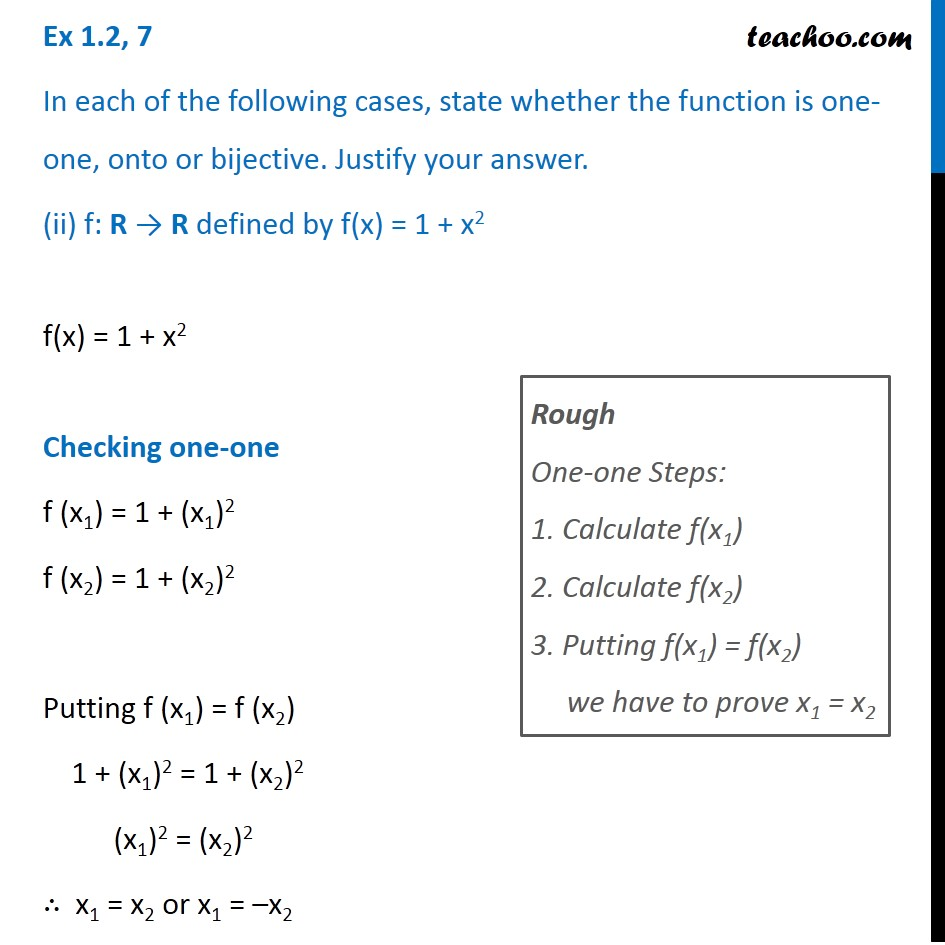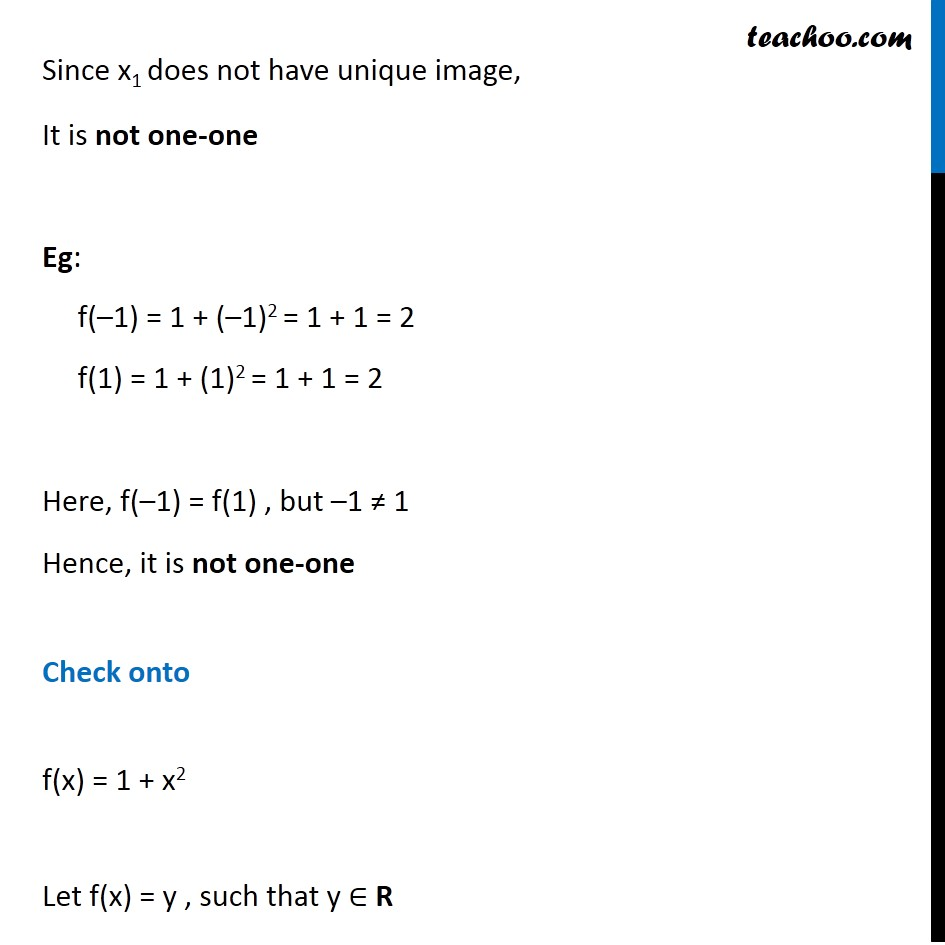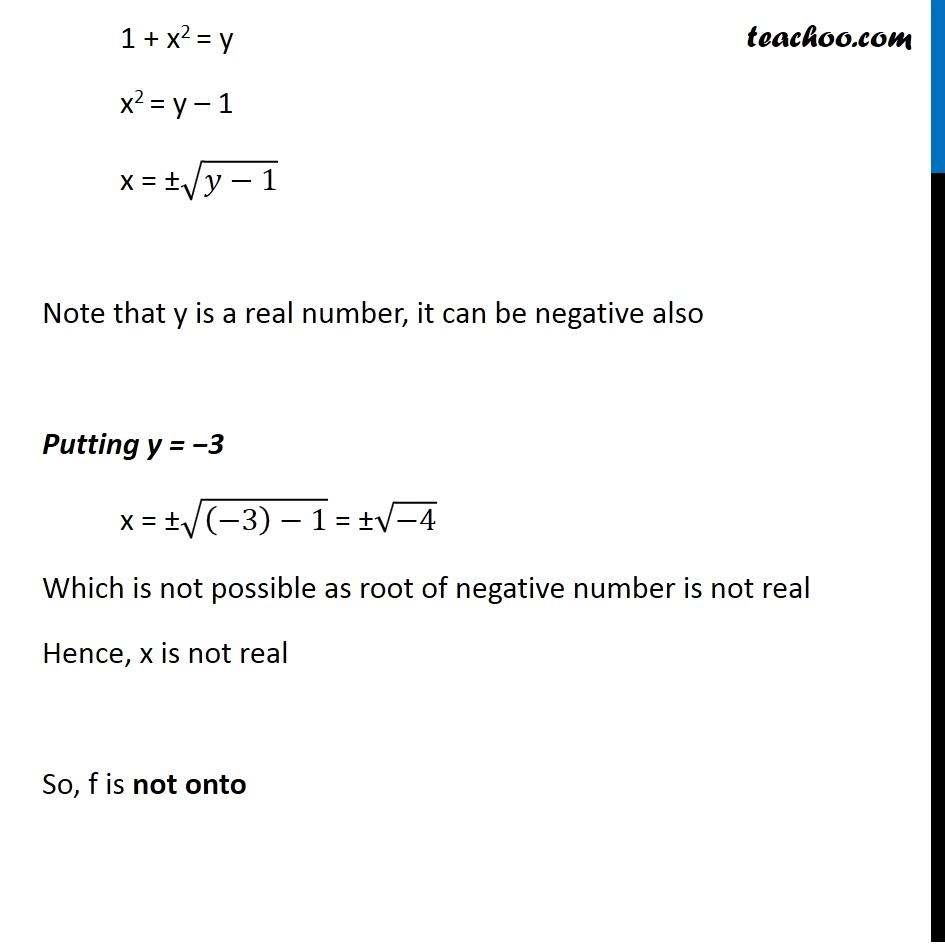Ex 1.2

Chapter 1 Class 12 Relation and Functions
Serial order wiseMaths Crash Course - Live lectures + all videos + Real time Doubt solving!

### Transcript

Ex 1.2, 7 In each of the following cases, state whether the function is one-one, onto or bijective. Justify your answer. (ii) f: R → R defined by f(x) = 1 + x2 f(x) = 1 + x2 Checking one-one f (x1) = 1 + (x1)2 f (x2) = 1 + (x2)2 Putting f (x1) = f (x2) 1 + (x1)2 = 1 + (x2)2 (x1)2 = (x2)2 ∴ x1 = x2 or x1 = –x2 Rough One-one Steps: 1. Calculate f(x1) 2. Calculate f(x2) 3. Putting f(x1) = f(x2) we have to prove x1 = x2 Since x1 does not have unique image, It is not one-one Eg: f(–1) = 1 + (–1)2 = 1 + 1 = 2 f(1) = 1 + (1)2 = 1 + 1 = 2 Here, f(–1) = f(1) , but –1 ≠ 1 Hence, it is not one-one Check onto f(x) = 1 + x2 Let f(x) = y , such that y ∈ R 1 + x2 = y x2 = y – 1 x = ±√(𝑦−1) Note that y is a real number, it can be negative also Putting y = −3 x = ±√((−3)−1) = ±√(−4) Which is not possible as root of negative number is not real Hence, x is not real So, f is not onto